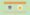# CBSE Class 11 Maths RS Aggarwal SolutionsClass 11 is also important for the students it makes the base for class 12. So, it is necessary that students must prepare thoroughly for the class 11 exam with the help of class 11 Maths Solutions textbooks. CBSE Class 11 Maths RS Aggarwal Solutions is one of the essential study materials for the students that want to score high marks.

CBSE Class 11 Maths RS Aggarwal Solutions are the most preferred study tool by students that wish to attain good marks in the exam. These Solutions are comprehensive and are created by the mathematics expert that assists the students to easily clear all their doubts.

Class 11 Maths RS Aggarwal Solutions are prepared according to the CBSE guidelines and have strong chances to score good marks in the exam. These Solutions include step-by-step explanations for each chapter.

## CBSE Class 11 Maths RS Aggarwal Solutions

Class 11 Maths RS Aggarwal Solutions are a valuable resources for exam preparation. These Solutions strengthen the foundation in the Maths subject for class 12 and also enable the students to tackle different types of questions and even difficult questions effortlessly. The students should download class 11 Maths RS Aggarwal Solutions PDF that make them to prepare for their final exam in a better way and to score high marks.

Class 11 Maths RS Aggarwal Solutions PDF download enables the students to attain a good academic score and enhance their problem-solving skills by solving the chapter-wise problems on a regular basis. RS Aggarwal Solutions is the best reference guide that assists the students to score good marks in the exam.

Class 11 Maths RS Aggarwal Solutions PDF are prepared in a step-wise manner that boosts the students’ exam preparation and also assists them to self analyze the weaker areas so that they can practice more to score good marks. The students can effortlessly download class 11 Maths RS Aggarwal Solutions and access it from any device, anywhere, and at any time.

Here, the students can download class 11 Maths RS Aggarwal Solutions chapter-wise:

• Chapter 1 – Sets
• Chapter 2 – Relations
• Chapter 3 – Functions
• Chapter 4 – Measurement of Angles
• Chapter 5 – Trigonometric Functions
• Chapter 6 – Graphs of Trigonometric Functions
• Chapter 7 – Trigonometric Ratios of Compound Angles
• Chapter 8 – Transformation Formulae
• Chapter 9 – Trigonometric Ratios of Multiple and Sub Multiple Angles
• Chapter 10 – Sine and Cosine Formulae and Their Applications
• Chapter 11 – Trigonometric Equations
• Chapter 12 – Mathematical Induction
• Chapter 13 – Complex Numbers
• Chapter 14 – Quadratic Equations
• Chapter 15 – Linear Inequations
• Chapter 16 – Permutations
• Chapter 17 – Combinations
• Chapter 18 – Binomial Theorem
• Chapter 19 – Arithmetic Progressions
• Chapter 20 – Geometric Progressions
• Chapter 21 – Some Special Series
• Chapter 22 – Brief Review of Cartesian System of Rectangular Coordinates
• Chapter 23 – The Straight Lines
• Chapter 24 – The Circle
• Chapter 25 – Parabola
• Chapter 26 – Ellipse
• Chapter 28 – Introduction To 3D Coordinate Geometry
• Chapter 29 – Limits
• Chapter 30 – Derivatives
• Chapter 31 – Mathematical Reasoning
• Chapter 32 – Statistics
• Chapter 33 – ProbabilityDownload Ultimate Study Materials to Boost Your Preparation CBSE Class 11 Maths Study Materials NCERT 11th Maths Books CBSE Guide Class 11th Class 11 goUnlimited Ebooks

## Benefits of CBSE Class 11 Maths RS Aggarwal Solutions

Class 11 Maths RS Aggarwal Solutions PDF include 33 chapters on topics such as sets, relations, functions, linear equations in one variable, combinations, binomial theorem, and more. Class 11 Maths RS Aggarwal Solutions are entirely based on the latest pattern of CBSE and are prepared by the experts.

Some notable benefits are as given below:

• RS Aggarwal Solutions are thoroughly researched and prepared by the experts.
• Class 11 Maths RS Aggarwal Solutions are comprehensive and provided in a simple and understandable language
• Class 11 Maths RS Aggarwal Solutions PDF include step-wise Solutions that enable the students to get to know the best approach to solve the problems of each chapter
• The students can develop problem-solving skills and can tackle any type of questions easily with the help of class 11 Maths RS Aggarwal Solutions
• The students can download class 11 Maths RS Aggarwal Solutions and also access in the online or offline mode as per their convenience

We have covered the detailed guide on CBSE Class 11 Maths RS Aggarwal Solutions. Feel free to ask any questions in the comment section below.